## Facebook Hacker Cup round 1 problem analysis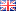Over the past 24 hours the first official round of the Facebook Hacker Cup was held, with thousands of qualified competitors competing for one of (at least) 500 spots to participate in round 2. There was a nice spread in topics for the problems and their difficulty was much more balanced than in the qualification round, which made for quite an enjoyable match with many competitors solving all three problems.

Like last week, I will analyze the problems in order of increasing difficulty. Spoilers abound, so don't read on if you want to try to solve the problems yourself!

#### Squished Status

Problem description here.

The problem is to compute in how many ways a string S can be split up into parts such that each part is an integer between and 1 and M. To calculate this, we could try all different ways of breaking off the first part (which, due to the limit on M, will be at most three digits long) and then calculating the number of combinations to split up the resulting suffix, yielding a recursive solution.

Explicitly generating all possible ways one by one will be too slow, as the fact that we must return the answer modulo 4-billion-something suggests. To generate an efficient solution we need to exploit the fact that we are only processing suffixes of the original string S, and the number of ways we can split up such a suffix is independent of the way we have split up the corresponding prefix. This allows us to eliminate a lot of redundant work.

Formally, let Si be the i-th character in S (starting from 0), L the length of S, Si,j the substring of S from the i-th to the j-th character (exclusive), and Ci the number of ways to split up Si,L (i.e. the suffix of S starting from the i-th character). Then we want to compute C0 and we can define Ci as the sum of all Cj for all j such that Si,j < M. There are two special cases: CL is 1 (since there is only one way to split up the empty string) and Ci=0 if Si=0 because we are not allowed to start numbers with leading zeroes.

To compute Ci we only need to know values of Cj for j > i, so we can easily compute C from back to front, starting with CL=1 and ending with C0, which is the desired answer. Programming contest veterans would immediately recognize this as an instance of dynamic programming which is a very useful technique for problems like this. The resulting algorithm runs in O(L×log M) time.

Note that the input file format is slightly tricky for this problem since input tokens may or may not be on the same line. The easiest way to deal with this is just split all input around whitespace. Apart from that, the implementation is straightforward:

Python:
 ```1 2 3 4 5 6 7 8 9 10 11 12 13 14 15 16 ``` ```from sys import stdin tokens = stdin.read().split() N = int(tokens.pop(0)) for n in range(1, N + 1): M, S = int(tokens.pop(0)), tokens.pop(0) L = len(S) C = *L +  for i in reversed(range(L)): if S[i] == '0': continue for j in range(i + 1, L + 1): if int(S[i:j]) > M: break C[i] += C[j] C[i] %= 0xfaceb00c print('Case #%d: %d'%(n, C))```

#### Recover the Sequence

Problem statement here.

We are asked to recover an unsorted sequence based on a trace of the merge sort algorithm run on the original input. This is daunting at first glance (partially due to the long problem statement) but it's actually quite easy, because re-playing the original execution of the algorithm (using the supplied execution trace to branch in the right directions) suffices to recover the original order of the elements.

For example, consider the sequence [3,1,2] which yields the trace "122". We don't know the original sequence but we can execute the algorithm starting with a list of three unknown variables, say [a,b,c]. Of course, we can't compare these variables, but fortunately we don't have to: the result of the comparisons is already given as part of the execution trace. So [a,b,c] is sorted into [b,c,a]. Since we know that the result must be a sorted sequence, we can equate [b,c,a]=[1,2,3] and that means the original sequence [a,b,c]=[3,1,2].

Implementing this approach is straightforward; most of the actual code sorting code can be adapted from the code given in the problem statement. In the end we have to calculate and print a checksum of the original sequence instead of the sequence itself. (To me, this seemed unnecessary considering these sequences are typically shorter than the execution traces that are given in the input.)

Python:
 ```1 2 3 4 5 6 7 8 9 10 11 12 13 14 15 16 17 18 19 20 21 22 23 24 25 26 27 28 29 30 31 32 33 34 35 36 37 38 39 40 41 42 43 44 ``` ```def merge_sort(arr): n = len(arr) if n <= 1: return arr mid = n//2 first_half = merge_sort(arr[0:mid]) second_half = merge_sort(arr[mid:n]) return merge(first_half, second_half) def merge(arr1, arr2): global pos result = [] i = j = 0 while i < len(arr1) and j < len(arr2): if S[pos] == '1': result.append(arr1[i]) i += 1 else: result.append(arr2[j]) j += 1 pos += 1 result.extend(arr1[i:]) result.extend(arr2[j:]) return result from sys import stdin for t in range(1, int(stdin.readline()) + 1): N = int(stdin.readline()) S = stdin.readline() # Re-execute algorithm: pos = 0 sorted = merge_sort(range(N)) # Recover the original sequence: sequence = *N for i in range(N): sequence[sorted[i]] = i + 1 # Compute the checksum checksum = 1 for i in range(N): checksum = (31*checksum + sequence[i])%1000003 print('Case #%d: %d'%(t, checksum))```

#### Checkpoint

Problem description here.

The last problem requires the most theoretical knowledge. Most of the work consists of computing (part of) Pascal's triangle though how that relates to the problem description is not immediately clear. Let's decompose the problem from top to bottom.

We are given a single number S, the total number of (shortest!) paths from start to finish that go through an intermediate point; we are free to place the points wherever we want to minimize our shortest path. That means S=AxB where A is the number of paths from the start to the intermediate point and B the number of paths from there to the finish. Let dist(n) be the minimal distance to a destination that has n distinct shortest paths leading to it. Then the answer is the minimum of dist(A)+dist(B) for all valid pairs of A and B, and since B=S/A (and vice versa) it suffices to test all divisors of S to find that minimum.

Now just need an efficient way to compute dist(n). We are looking for a destination (x,y) such that x+y (the distance to the destination) is minimal and the number of ways to reach it equals n. So how many ways are there to reach (x,y)? Any shortest path necessarily consists of x moves to the right and y moves to the top, and there are exactly C(x+y,x) (or equivalently, C(x+y,y)) ways to order those moves. Here, C(n,m) is the choose function which calculates how many combinations exist for selecting m elements from a group of n.

For those familiar with combinatorics, the relationship with Pascal's triangle should now be clear, as C(n,m) equals the m-th element in the n-th row of Pascal's triangle (if we count starting from 0). Therefore dist(n) can be defined as the index of the first row in Pascal's triangle where the number n appears.

We need to be able to compute values of dist(n) for n between 1 and S (inclusive), but computing ten million rows of Pascal's triangle would take too much time. Fortunately, we can exploit the horizontal symmetry of the triangle to evaluate only the part of the triangle that contains numbers up to S. For example, the image below shows that if we only care about values up to 6, we can ignore most of the bottom part of the triangle: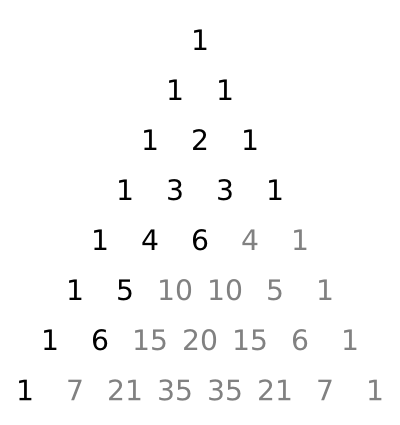This shows that although the area of the full triangle is very large, we are only need to look at a shallow part of it to find the values up to S that we are interested in. To speed up things even more, we can take advantage of the fact that row i always starts with values 1, i, .. so we only need to concern ourselves with the computation of values starting from the third element in each row, and we can stop as soon as that element exceeds S.

Now that we have done all the necessary thinking to solve this problem efficiently, we can start implementing. First, precompute the values of dist(x) which are found in Pascal's triangle for x≤107 (the maximum possible value of S), then loop over the possible divisors and try which one yields the optimal solution.

Preprocessing takes O(sqrt S × log S) time and then each testcase can be solved in O(sqrt(S)) time. edit: I updated this analysis and the corresponding code after a nice discussion with Robin Lee about the efficiency of my approach in the comments.

Python:
 ```1 2 3 4 5 6 7 8 9 10 11 12 13 14 15 16 17 18 19 20 21 22 23 24 25 26 27 28 29 30 31 ``` ```limit = 10**7 # Generate (the relevant part of) Pascal's triangle dist = {} row = [1,2,1] n = 2 while row <= limit: # Update shortest distances: for c in row[2:]: if n < dist.get(c, c): dist[c] = n # Calculate (relevant part of) next_row row: next_row =  for i in range(n): c = row[i] + row[i + 1] next_row.append(c) if c > limit: break else: next_row.append(1) row = next_row n += 1 from sys import stdin for r in range(1, int(stdin.readline()) + 1): S = int(stdin.readline()) answer = min( dist.get(i, i) + dist.get(S//i, S//i) for i in range(1, int(S**.5) + 1) if S%i == 0 ) print('Case #%d: %d'%(r, answer))```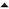06-'12 Using netcat to build a simple TCP proxy in Linux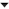01-'12 Facebook Hacker Cup qualification round problem analysis

Ok. Ik ben hier dus echt te lomp voor. Ik snap gewoon geen donder van de uitleg...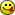The presented solution isn't optimal, as the binomial coefficients do not have to be precalculated (they can be worked out at runtime using binary search).

Robin: I don't see how you can binary-search for binomial coefficients. Could you elaborate on this?

There's an error in the solution of squished status (the solve function is not defined).

skinny wrote on Sunday 29 January 2012 @ 22:42:
There's an error in the solution of squished status (the solve function is not defined).
Whoops, you're right. That expression should just be C (like explained in the text).

Robin Lee wrote on Sunday 29 January 2012 @ 21:40:
The presented solution isn't optimal, as the binomial coefficients do not have to be precalculated (they can be worked out at runtime using binary search).
I'm curious as to how this would be possible, I was looking for a similar approach but failed to find a function/algorithm for it

I say, check http://projecteuler.net/.
All sorts of problems like the one you presented.

Sorry chaps (Soultaker and Tharulez specifically), I didn't get any email notification when you replied to me.

Solutions are now available, please have a look at my code. I'm the only Robin on the first page, alternatively check out http://pastebin.com/A4Eu4xka. Alternatively it's using an idea from the solution to a problem from another programming contest, NWERC 2011 Problem A (Binomial Coefficients)

Is this written by native English speakers? I don't understand the following sentence at all, from the "SQUISHED STATUS" problem, someone care to elaborate?

"Due to database corruption, a compressed status may contain sequences of digits that could not result from removing the spaces in an encoded status message"

Robin Lee wrote on Monday 30 January 2012 @ 08:04:
Alternatively it's using an idea from the solution to a problem from another programming contest, NWERC 2011 Problem A (Binomial Coefficients)
If I understand this right, you are looping over all relevant values of k up to sqrt(x) or so and then binary search in the corresponding diagonal to find the value of n that gets you closest to the desired result.

That's an interesting approach, so thank you for posting! I'm not entirely sure the approach is inherently more efficient, though.

The sqrt(x) bound comes from not considering the first two diagonals (which contain only 1s and the natural numbers respectively) since the third diagonal contains the triangular numbers. We can use the same trick to optimize my solution by leaving the dictionary uninitialized. This reduces the preprocessing time to O(sqrt(S)×log S) (since entries in each row grow exponentially fast) and we still need only O(1) time per divisor (or O(sqrt S) per test case).

This should already be pretty close to optimal, but in principle we could ignore the third diagonal too (and check whether each divisor is a triangular number on the fly) and then time required to generate divisors dominates the preprocessing time, for an O(sqrt S) solution. I don't think that's worth the effort, though.

[Comment edited on maandag 30 januari 2012 16:05]